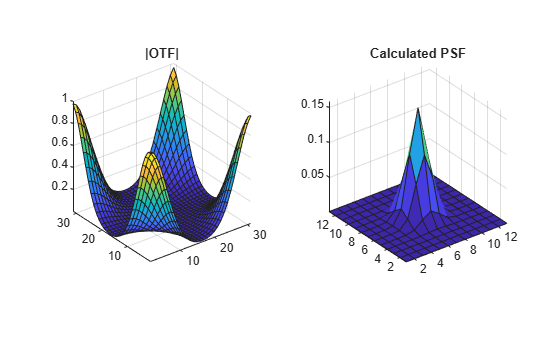otf2psf

Convert optical transfer function to point-spread function

Description

example

PSF = otf2psf(OTF) computes the inverse Fast Fourier Transform of the optical transfer function (OTF) and creates a point-spread function (PSF), centered at the origin.

PSF = otf2psf(OTF,sz) specifies the size, sz, of the output point-spread function.

Examples

collapse all

PSF  = fspecial('gaussian',13,1);

Convert the PSF to an Optical Transfer Function (OTF).

OTF  = psf2otf(PSF,[31 31]);

Convert the OTF back to a PSF.

PSF2 = otf2psf(OTF,size(PSF));

Plot the PSF and the OTF.

subplot(1,2,1)
surf(abs(OTF))
title('|OTF|');
axis square
axis tight
subplot(1,2,2)
surf(PSF2)
title('Corresponding PSF');
axis square
axis tightInput Arguments

collapse all

Optical transfer function, specified as a numeric array of any dimension.

Data Types: single | double | int8 | int16 | int32 | int64 | uint8 | uint16 | uint32 | uint64
Complex Number Support: Yes

Size of the output point-spread function PSF, specified as a vector of positive integers. The size of PSF must not exceed the size of OTF in any dimension. By default, PSF is the same size as OTF.

Data Types: double

Output Arguments

collapse all

Point-spread function, centered at the origin, returned as a numeric array of size sz.

Data Types: double
Complex Number Support: Yes

Tips

• To center the PSF at the origin, otf2psf circularly shifts the values of the output array down (or to the right) until the (1,1) element reaches the central position, then it crops the result to match dimensions specified by sz.

• This function is used in image convolution and deconvolution when the operations involve the FFT.